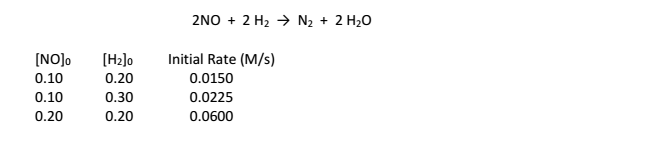# Problem: Initial rate data have been determined at a certain temperature for the gaseous reaction. The numerical value of the rate constant is: A. 7.5 B. 3.0 x 10 -3 C. 380 D. 0.75 E. 3.0 x 10 -4

###### FREE Expert Solution
81% (383 ratings)
###### Problem Details

Initial rate data have been determined at a certain temperature for the gaseous reaction.

The numerical value of the rate constant is:

A. 7.5

B. 3.0 x 10 -3

C. 380

D. 0.75

E. 3.0 x 10 -4What scientific concept do you need to know in order to solve this problem?

Our tutors have indicated that to solve this problem you will need to apply the Rate Law concept. You can view video lessons to learn Rate Law. Or if you need more Rate Law practice, you can also practice Rate Law practice problems.

What is the difficulty of this problem?

Our tutors rated the difficulty ofInitial rate data have been determined at a certain temperat...as high difficulty.

How long does this problem take to solve?

Our expert Chemistry tutor, Dasha took 7 minutes and 3 seconds to solve this problem. You can follow their steps in the video explanation above.

What professor is this problem relevant for?

Based on our data, we think this problem is relevant for Professor Co's class at OSU.